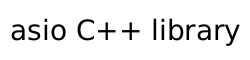### Endpoint requirements

A type `X` meets the `Endpoint` requirements if it satisfies the requirements of `Destructible` (C++Std [destructible]), `DefaultConstructible` (C++Std [defaultconstructible]), `CopyConstructible` (C++Std [copyconstructible]), and `CopyAssignable` (C++Std [copyassignable]), as well as the additional requirements listed below.

The default constructor and move operations of the type `X` shall not exit via an exception.

In the table below, `a` denotes a (possibly const) value of type `X`, and `u` denotes an identifier.

Table 14. Endpoint requirements

expression

type

assertion/note
pre/post-conditions

`X::protocol_type`

type meeting `Protocol` requirements

`a.protocol()`

`protocol_type`

In the table below, `a` denotes a (possibly const) value of type `X`, `b` denotes a value of type `X`, and `s` denotes a (possibly const) value of a type that is convertible to `size_t` and denotes a size in bytes.

Table 15. Endpoint requirements for extensible implementations

expression

type

assertion/note
pre/post-conditions

`a.data()`

`const void*`

Returns a pointer suitable for passing as the address argument to functions such as POSIX `connect()`, or as the dest_addr argument to functions such as POSIX `sendto()`. The implementation shall perform a `static_cast` on the pointer to convert it to ```const sockaddr*```.

`b.data()`

`void*`

Returns a pointer suitable for passing as the address argument to functions such as POSIX `accept()`, `getpeername()`, `getsockname()` and `recvfrom()`. The implementation shall perform a `static_cast` on the pointer to convert it to `sockaddr*`.

`a.size()`

`size_t`

Returns a value suitable for passing as the address_len argument to functions such as POSIX `connect()`, or as the dest_len argument to functions such as POSIX `sendto()`, after appropriate integer conversion has been performed.

`b.resize(s)`

pre: ```s >= 0```
post: ```a.size() == s```
Passed the value contained in the address_len argument to functions such as POSIX `accept()`, `getpeername()`, `getsockname()` and `recvfrom()`, after successful completion of the function. Permitted to throw an exception if the protocol associated with the endpoint object `a` does not support the specified size.

`a.capacity()`

`size_t`

Returns a value suitable for passing as the address_len argument to functions such as POSIX `accept()`, `getpeername()`, `getsockname()` and `recvfrom()`, after appropriate integer conversion has been performed.